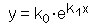The SDL Component Suite is an industry leading collection of components supporting scientific and engineering computing. Please visit the SDL Web site for more information....## CalcExponentialFitUnit: SDL_math2 Class: Declaration: procedure CalcExponentialFit (var k0, k1, FitQual: double);

The procedure CalcExponentialFit calculates the best fitting exponential curve for a given set of data. The curve is determined by the equationThe values of x and y are given by the data samples, the parameters k0 and k1 are estimated by CalcExponentialFit using a least squares approximation. An exception is raised if the fit cannot be calculated (i.e. if the x-values are too large).

The data points [x,y] have to be entered using the routine EnterStatValue. A minimum number of 3 values is required in order to apply CalcExponentialFit. Do not forget to reset the statistics calculation before entering any new data sets (use the method Init).

In addition to the parameters k0 and k1, CalcExponentialFit calculates the quality of fit FitQual. This parameter may vary between 0.0 and 1.0, indicating the best possible fit if FitQual equals 1.0.

 Hint 1: The quality of fit calculated by CalcExponentialFit is not adjusted for the degree of freedoms in the regression parameters.

 Hint 2: The regression routine requires both the x- and the y-values to be greater than zero. The curve fitting algorithm raises an exception in the case of negative or zero values. In the case you have exclusively negative y-values you can apply the regression algorithm by changing the sign of all y-values before performing the regression. As a consequence you have to change the sign of the estimated parameter k0 as well.

Last Update: 2012-Oct-20